# Samacheer Kalvi Books: Tamilnadu State Board Text Books Solutions

## Samacheer Kalvi 11th Computer Science Chapter 7 Composition and Decomposition Notes PDF Download: Tamil Nadu STD 11th Computer Science Chapter 7 Composition and Decomposition NotesSamacheer Kalvi 11th Computer Science Chapter 7 Composition and Decomposition Notes PDF Download: Tamil Nadu STD 11th Computer Science Chapter 7 Composition and Decomposition Notes

## Samacheer Kalvi 11th Computer Science Chapter 7 Composition and Decomposition Notes PDF Download

We bring to you specially curated Samacheer Kalvi 11th Computer Science Chapter 7 Composition and Decomposition Notes PDF which have been prepared by our subject experts after carefully following the trend of the exam in the last few years. The notes will not only serve for revision purposes, but also will have several cuts and easy methods to go about a difficult problem.

 Board Tamilnadu Board Study Material Notes Class Samacheer Kalvi 11th Computer Science Subject 11th Computer Science Chapter Chapter 7 Composition and Decomposition Format PDF Provider Samacheer Kalvi Books

## How to Download Samacheer Kalvi 11th Computer Science Chapter 7 Composition and Decomposition Notes PDFs?

2. Click on the Samacheer Kalvi 11th Computer Science Notes PDF.
3. Look for your preferred subject.
4. Now download the Samacheer Kalvi 11th Computer Science Chapter 7 Composition and Decomposition notes PDF.

## Download Samacheer Kalvi 11th Computer Science Chapter 7 Composition and Decomposition Chapterwise Notes PDF

PART – 1

Question 1.
Suppose u, v = 10, 5 before the assignment. What are the values of u and v after the sequence of assignments?
1. u : = v
2. v : = u
(a) u, v = 5, 5
(b) u, v = 10, 5
(c) u, v = 5, 10
(d) u, v = 10, 10
(a) u, v = 5, 5

Question 2.
Which of the following properties is true after the assignment (at line 3?
1. – – i + j = 0
2. i, j : = i + 1, j – 1
3. – – ?
(a) i + j > 0
(b) i + j < 0
(c) i + j = 0
(d) i = j
(c) i + j = 0

Question 3.
If C1 is false and C2 is true, the compound statement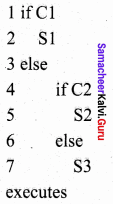(a) S1
(b) S2
(c) S3
(d) none
(b) S2

Question 4.
If C is false just before the loop, the control flows through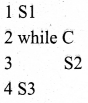(a) S1 ; S3
(b) S1 ; S2 ; S3
(c) S1 ; S2 ; S2 ; S2 ; S3
(d) S1 ; S2 ; S2 ; S2 ; S3
(a) S1 ; S3

Question 5.
If C is true, S1 is executed in both the flowcharts, but S2 is executed in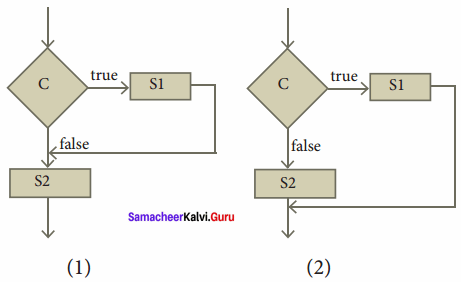(a) (1) only
(b) (2) only
(c) both (1) and (2)
(d) neither (1) nor (2)
(a) (1) only

Question 6.
How many times the loop is iterated?
i : = 0
while i ≠ 5
i : = i + 1
(a) 4
(b) 5
(c) 6
(d) 0
(b) 5

PART – 2

Question 1.
Distinguish between a condition and a statement.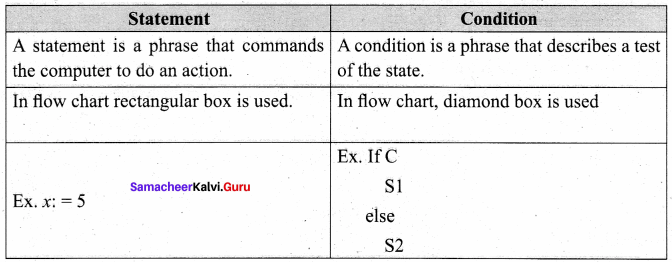Question 2.
Draw a flowchart for a conditional statement.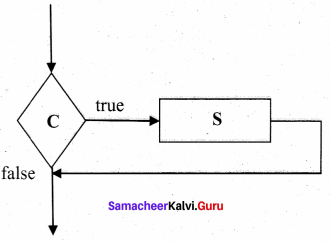Question 3.
Both conditional statements and iterative statements have a condition and a statement. How do they differ?

• A conditional statement is executed only if the condition is true. Otherwise, nothing is done.
• An iterative statement repeatedly evaluates a condition and executes a statement as long as the condition is true.

Question 4.
What is the difference between an algorithm and a program?
Algorithm:

• An algorithm is for human readers to understand.
• Knowledge of English is needed.
• Easy to understand.

Program:

• The program is for the computers to execute directly.
• Knowledge of programming language is required.
• It is difficult to understand.

Question 5.
Why is a function an abstraction?
A function is like a sub-algorithm. A function is specified by its own input property, and the desired input-output relation. So, other things are not required. So it is abstract.

Question 6.
How do we refine a statement?
In a refinement, each statement is repeatedly expanded into more detailed statements in the subsequent levels.

PART – 3
Explain in Brief

Question 1.
For the given two flowcharts write the pseudo code.

1. Enter A,B
2. Initialise Q = 0, r = A
3. If r > B, then do Q = Q + 1; r = r – B else r, q
4. Exit

Question 2.
If C is false in line 2, trace the control flow in this algorithm.

1. S1
2. – – C is false
3. if C
4. S2
5. else
6. S3
7. S4

S1 ; S2 ; S4

Question 3.
What is case analysis?
Case analysis splits the problem into an exhaustive set of disjoint cases. For each case, the problem is solved independently.
If C1, C2, and C3 are conditions, and S1, S2, S3 and S4 are statements, a 4-case analysis statement has the form,
i) case C1
ii) S1
iii) case C2
iv) S2
v) case C3
vi) S3
vii) else
viii) S4

Question 4.
Draw a flowchart for -3 case analysis using alternative statements.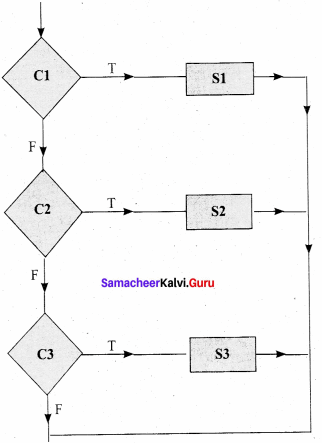Question 5.
Define a function to double a number in two different ways:

1. n + n
2. 2 x n

Function to double a number in a first way using d = n + n
i) double(n)
ii) – – inputs: n is a real number
iii) – – outputs : d is a real number
d = n +n
Function to double a number in first way using d = 2 x n
i) double(n)
ii) — inputs: n is a real number
iii) – – outputs : d is a real number
d = 2 x n

PART – 4
IV. Explain in Detail

Question 1.
Exchange the contents: Given two glasses marked A and B. Glass A is full of apple drink and glass B is full of grape drink. Write the specification for exchanging the contents of glasses A and B, and write a sequence of assignments to satisfy the specification.
Let the variables a, b, c represent Glass A, Glass B and Glass C and a, b, c can store values APPLE, GRAPE or EMPTY.
Specification:

1. exchange (a, b)
2. – – inputs: a, b : = APPLE, GRAPE
3. – – outputs: a, b : = GRAPE, APPLE

Algorithm: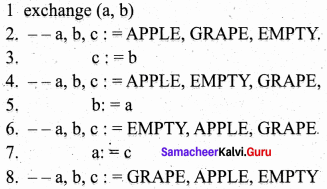Question 2.
Circulate the contents: Write the specification and construct an algorithm to circulate the contents of the variables A, B, and C as shown below: The arrows indicate that B gets the value of A, C gets the value of B and A gets the value of C.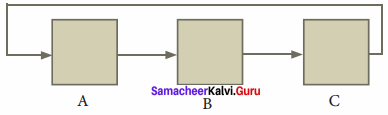Specifications:

1. circulate
2. – – inputs: a, b, c: = A, B, C
3. – – outputs: a, b, c: = C, A, B

Algorithm:

1. circulate (a, b, c)
2. – – a, b, c: = A, B, C
3. temp : = c
4. c : = b
5. b : = a
6. a : = temp
7. – – a, b, c: = C, A, B

Question 3.
Decanting problem. You are given three bottles of capacities 5, 8, and 3 liters. The 8L bottle is filled with oil, while the other two are empty. Divide the oil in an 8L bottle into two equal quantities. Represent the state of the process by appropriate variables. What are the initial and final states of the process? Model the decanting of oil from one bottle to another by assignment. Write a sequence of assignments to achieve the final state.
(a)
Let a, b, c be the variables whose maximum values are 8L, 5L, and 3L respectively.
Initial State: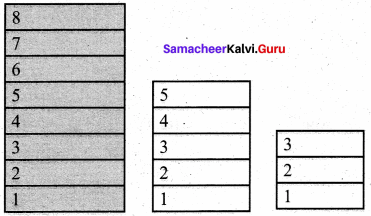a, b, c: = 8, 0, 0

Final State: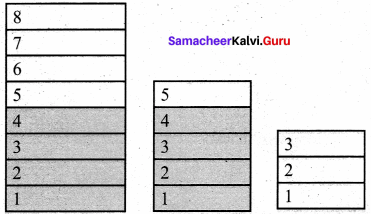a, b, c: = 4, 4, 0

Specifications:

1. decant
2. – – inputs: a, b, c : = 8, 0, 0
3. – – outputs: a, b, c : = 4, 4, 0

Algorithm:
Let us assume that a: = b denotes oil in b is poured into a bottle until either “a” is full or “b” becomes empty.

1. decant (a, b, c)
2. – – a, b, c : = 8, 0, 0
3. b : = a
4. – – a, b, c : = 3, 5, 0
5. c : = b
6. – – a, b, c : = 3, 2, 3
7. a : = c
8. – – a, b, c : = 6, 2, 0
9. c : = b
10. – – a, b, c : = 6, 0, 2
11. b : = a
12. – – a, b, c : = 1, 5, 2
13. c : = b
14. – – a, b, c : = 1, 4, 3
15. a : = c
16. – – a, b, c: = 4, 4, 0

Question 4.
Trace the step-by-step execution of the algorithm for factorial(4).
factorial(n)
– – inputs: n is an integer, n ≥ 0
– – outputs : f = n!
f, i : = 1,1
while i ≤ n
f, i : = f x i, i + 1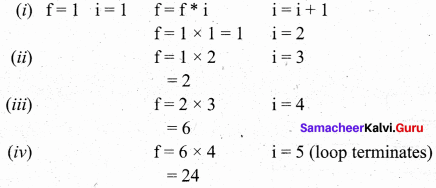### Samacheer Kalvi 11th Computer Science Composition and Decomposition Additional Questions and Answers

PART – 1

Question 1.
How many different notations are there for representing algorithms?
a) 5
b) 4
c) 3
d) 2
c) 3

Question 2.
………………. is a diagrammatic notation for representing algorithms.
(a) Pseudocode
(b) Flowchart
(c) Program
(d) Languages
(b) Flowchart

Question 3.
A _________ is a notation for expressing algorithms to be executed by computers.
a) programming language
b) pseudo code
c) flow chart
d) none of these
a) programming language

Question 4.
A ………………. statement is composed of a sequence of statements.
(a) iterative
(b) conditional
(c) sequential
(d) alternative
(c) sequential

Question 5.
_________ expressed in pseudo-code are not intended to be executed by computers.
a) programming language
b) algorithm
c) flow chart
d) None of these
b) algorithm

Question 6.
The triangle is right-angled, if ……………….
(a) C = a – b
(b) C2 = a2 + b2
(c) C2 = (a + b)2
(d) c2 = a2 – b2
(b) C2 = a2 + b2

Question 7.
_________ give a visual intuition of the flow of control when the algorithm is executed.
a) programming language
b) pseudo-code
c) flow chart
d) None of these
c) flow chart

Question 8.
After an algorithmic problem is decomposed into subproblems, we can abstract the subproblems as ……………….
(a) refinement
(b) pseudo – code
(c) decomposition
(d) functions
(d) functions

Question 9.
_________ is an example of programming language.
a) C b) C++
c) Python
d) All the above
d) All the above

Question 10.
Which one of the following has only high-level details?
(a) Flow chart
(b) Algorithm
(c) Programs
(d) Pseudocode
(b) Algorithm

Question 11.
_________ must obey the grammar of the programming language exactly.
a) programs
b) pseudo-code
c) flow charts
d) None of these
a) programs

Question 12.
Which one of the following notations will be executed by computers?
(a) Flow chart
(b) Pseudocode
(c) Programming languages
(d) Compiler
(c) Programming languages

Question 13.
There is a _________ which translates the program into instructions executable by the computer.
a) translator
b) convertor
c) transcriptor
d) None of these
a) translator

Question 14.
Which one of the following algorithmic notations is used for giving visual intuition of control flow?
(a) Flow chart
(b) Programming languages
(c) Pseudocode
(d) Compiler
(a) Flow chart

Question 15.
_________ uses the same building blocks as programs, such as variables and control flow.
a) programs
b) pseudo-code
c) flow charts
d) None of these
b) pseudo-code

Question 16.
Identify the statement which is wrong ……………….
(a) Programs must obey the grammar of the Programming language exactly.
(b) The punctuations in Programming language must be exactly.
(c) The Programming language is informal.
(d) Translator translates the programs into instructions.
(c) The Programming language is informal.

Question 17.
_________ is the most widely used notation to represent algorithms.
a) programs
b) pseudo-code
c) flow charts
d) None of these
b) pseudo-code

Question 18.
Identify the correct statement from the following?
(a) Pseudocode can be executed by the computer directly.
(b) Pseudocode cannot be executed by the computer directly.
(c) Algorithm cannot be expressed in Pseudocode
(d) Algorithm are written only for python language
(b) Pseudocode cannot be executed by the computer directly.

Question 19.
In flowcharts, _________ boxes represent simple statements.
a) arrows
b) diamond-shaped
c) rectangular shaped
d) None of these
c) rectangular shaped

Question 20.
Identify the correct statements.
(i) Pseudocode uses natural English for statements and conditions.
(ii) Pseudocode notation is a formal one.
(iii) There is no need to follow the rules of the Programming language grammar
(iv) It must be rigorous and correct.
(a) (i) (ii) (iii)
(b) (ii) (iii) (iv)
(c) (i) (iii) (iv)
(d) (i) (ii) (iii) (iv)
(c) (i) (iii) (iv)

Question 21.
In flowcharts, _________ describe how the control flows during the execution of the algorithm.
a) arrows
b) diamond-shaped
c) rectangular shaped
d) None of these
a) arrows

Question 22.
Find the correct flow chart diagram from the following.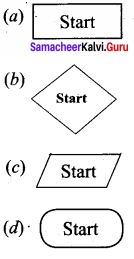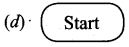Question 23.
In flowchart,_________ boxes represent inputs given and outputs produced.
a) arrows
b) diamond shaped
c) rectangular shaped
d) Parallelogram
d) Parallelogram

Question 24.
The symbol used for representing the box to be executed next is ………………..
(a) ⇒
(b) ⇓
(c) ↓
(d) ++
(c) ↓

Question 25.
_________ is a disadvantage of a flowchart.
a) Flowcharts are less compact than the representation of algorithms in a programming language or pseudo code.
b) They obscure the basic hierarchical structure of the algorithms.
c) Alternative statements and loops are disciplined control flow structures.
d) All the above
d) All the above

Question 26.
In the flow chart, rectangular boxes represent………………..
(a) statements
(b) condition
(c) Input
(d) End
(a) statements

Question 27.
Statements may be composed of other statements, leading to a _________ structure
of algorithms.
a) bottom-up
b) top-down
c) random
d) hierarchical
d) hierarchical

Question 28.
A collection of boxes containing statements and conditions connected by the arrow are ………………..
(a) compiler
(b) Flow chart
(c) Pseudocode
(d) Algorithm
(b) Flow chart

Question 29.
Match the following: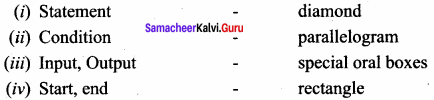(a) (i) -4 (ii) 0 – 1 (iii) – 2 (iv) – 3
(b) (i) – 1 (ii) – 2 (iii) – 3 (iv) – 4
(c) (i) – 4 (ii) – 2 (iii) – 1 (iv) – 3
(d) (i) – 3 (ii) – 2 (iii) – 1 (iv) – 4
(a) (i) -4 (ii) 0 – 1 (iii) – 2 (iv) – 3

Question 30.
There are __________ important control flow statements.
a) four
b) three
c) two
d) no
b) three

Question 31.
Statements composed of other statements are known as:
(a) Simple Statements
(b) Compound Statements
(c) Conditional
(d) Control flow
(b) Compound Statements

Question 32.
A __________ is a phrase that describes a test of the state.
a) condition
b) loop
c) structure
d) None of these
a) condition

Question 33.
Which one of the following statements is used to alter the normal flow of control of the program?
(a) Assignments
(b) Control flow
(c) Compound
(d) both b & c
(d) both b & c

Question 34.
In case analysis, the cases are __________ .
a) exhaustive
b) disjoint
c) A or B
d) none of these
c) A or B

Question 35.
Alternative statements analyze the problem into ……………….. cases.
(a) 2
(b) 3
(c) 4
(d) 5
(a) 2

Question 36.
The iterative statement is commonly known as a __________
a) loop
b) block
c) sequential
d) None of these
a) loop

Question 37.
If sC is a ……………….. statement.
(a) Conditional
(b) Alternative
(c) Case Analysis
(d) Iterative
(a) Conditional

Question 38.
__________ involves breaking down a problem into smaller and more manageable problems.
a) Composition
b) Decomposition
c) Eliminating
d) None of these
b) Decomposition

Question 39.
The iterative statement is commonly known as a ………………..
(a) loop
(b) Case Analysis
(c) Alternative
(d) Conditional
(a) loop

Question 40.
We can abstract the subproblems as__________
a) functions
b) arrays
c) structures
d) None of these
a) functions

PART – 2

Question 1.
What is a Programming Language?

1. A programming language is a notation for expressing algorithms to be executed by computers.
2. Programs must obey the grammar of the programming language exactly. Ex. C, C++, python.

Question 2.
Pseudocode is a notation similar to programming languages. Algorithms expressed in pseudo-code are not intended to be executed by computers, but for communication among people.

Question 3.
What is a Flow chart?
A flow chart is a diagrammatic notation for representing algorithms. A flow chart is a collection of boxes containing statements and conditions which are connected by arrows showing the order in which the boxes are to be executed.

Question 4.
List some of the programming languages.
The following are some of the programming languages.

1. C
2. C++
3. Python
4. Java

Question 5.
What is meant by Decomposition?
Decomposition is one of the elementary problem-solving techniques. An algorithm may be broken into parts, expressing only high-level details. Then, each part may be refined into smaller parts, expressing finer details or each part may be abstracted as a function.

Question 6.
What are the benefits of pseudocode?

• This notation is not formal nor exact.
• It uses the same building blocks as programs, such as variables and control flow.
• It allows the use of natural English for statements and conditions.
• there is no need to follow the rules of the grammar of a programming language.

Question 7.
Write the algorithm specification to find a minimum of 2 numbers.
Minimum (a, b)
– – inputs : a, b
– – outputs : result = a >1 b

Question 8.
Draw the flowchart for alternative control flow.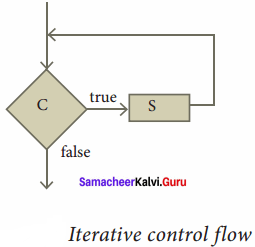PART – 3
III. Explain in Brief

Question 1.
Draw the diagram flowchart for integer division.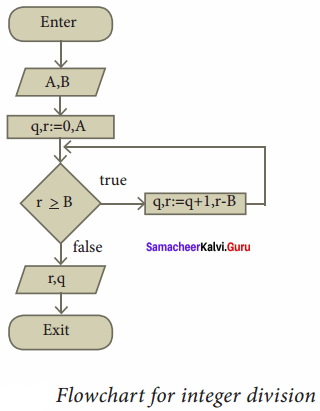Question 2.
Explain the conditional statement.
Conditional statement: Sometimes we need to execute a statement only if a condition is true and do nothing if the condition is false. This is equivalent to the alternative statement in which the else clause is empty. This variant of the alternative statement is called a conditional statement. If C is a condition and S is a statement, then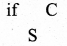is a statement, called a conditional statement, that describes the following action:

1. Test whether C is true or false.
2. If C is true then do S; otherwise do nothing.

The conditional control flow is depicted in the flowchart.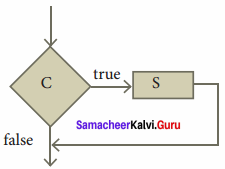Question 3.
Write an algorithm for finding the minimum of two numbers.
Algorithm minimum can be defined as
i) minimum(a, b)
ii) – – a, b
iii) if a < b
iv) result: = a
v) else
vi) result = b
vii) — result = a ↓ b.

Question 4.
Draw various symbols used in the flowchart with their usage.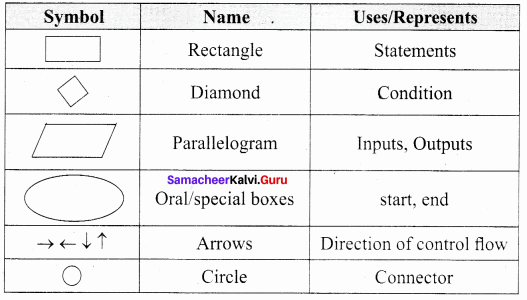Question 5.
Write an Algorithm to find a minimum of 2 numbers.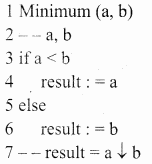Question 6.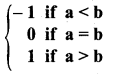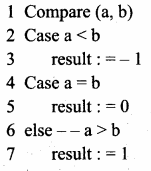Question 7.
Explain the Refinement process.
Each subproblem can be expanded into more detailed steps. Each step can be further expanded to still finer steps, and so on. This is known as refinement.

We can also abstract the subproblem. We specify each subproblem by its input property and the input-output relation. While solving the main problem, we only need to know the specification of the subproblems. We do not need to know how the subproblems are solved.

PART – 4
IV. Explain in Detail

Question 1.

1. Flowcharts are less compact than the representation of algorithms in a programming language or pseudo code.
2. They obscure the basic hierarchical structure of the algorithms.
3. Alternative statements and loops are disciplined control flow structures.

Question 2.
Explain the sequential statement in detail with the diagram.
A sequential statement is composed of a sequence of statements. The statements in the sequence are executed one after another, in the same order as they are written in the algorithm, and the control flow is said to be sequential. Let S1 and S2 be statements. A sequential statement composed of S1 and S2 is written as
S1
S2
In order to execute the sequential statement, first, do S1 and then do S2. The sequential statement given above can be represented in a flowchart. The arrow from S1 to S2 indicates that S1 is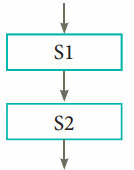Let the input property be P, and the input-output relation be Q, for a problem. If statement S solves the problem, it is written as

1. – – p
2. S
3. – – Q

If we decompose the problem into two components, we need to compose S as a sequence of two statements S1 and S2 such that the input-output relation of S1, say R, is the input property of S2.

1. – – P
2. S1
3. – – R
4. S2
5. – – Q

Question 3.
Explain Case analysis with suitable examples.
Case analysis
An alternative statement analyses the problem into two cases. The case analysis statement generalizes it to multiple cases.
Case analysis splits the problem into an exhaustive set of disjoint cases. For each case, the problem is solved independently.
If C1, C2, and C3 are conditions, and S1, S2, S3, and S4 are statements, a 4-case analysis statement has the form,
case C1
S1
case C2
S2
case C3
S3
Else
S4

The conditions C1, C2, and C3 are evaluated in turn. For the first condition that evaluates to true, the corresponding statement is executed, and the case analysis statement ends. If none of the conditions evaluates to true, then the default case S4 is executed.

• The cases are exhaustive: at least one of the cases is true. If all conditions are false, the default case is true.
• The cases are disjoint: only one of the cases is true. Though it is possible for more than one condition to be true, the case analysis always executes only one case, the first one that is true. If the three conditions are disjoint, then the four cases are (1) C1, (2) C2, (3) C3, (4) (not C1), and (not C2) and (not C3).

Question 4.
What is a function? Explain in detail.
After an algorithmic problem is decomposed into subproblems, we can abstract the subproblems as functions. A function is like a sub-algorithm. Similar to an algorithm, a function is specified by the input property and the desired input-output relation.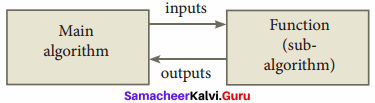To use a function in the main algorithm, the user needs to know only the specification of the function – the function name, the input property, and the input-output relation. The user must ensure that the inputs passed to the function will satisfy the specified property and can assume that the outputs from the function satisfy the input-output relation. Thus, users of the function need only to know what the function does, and not how it is done by the function. The function can be used a “black box” in solving other problems.

Ultimately, someone implements the function using an algorithm. However, users of the function need not know about the algorithm used to implement the function. It is hidden from the users. There is no need for the users to know how the function is implemented in order to use it.

An algorithm used to implement a function may maintain its own variables. These variables are local to the function in the sense that they are not visible to the user of the function. Consequently, the user has fewer variables to maintain in the main algorithm, reducing the clutter of the main algorithm.

Question 5.
Draw the flowchart for Eat breakfast.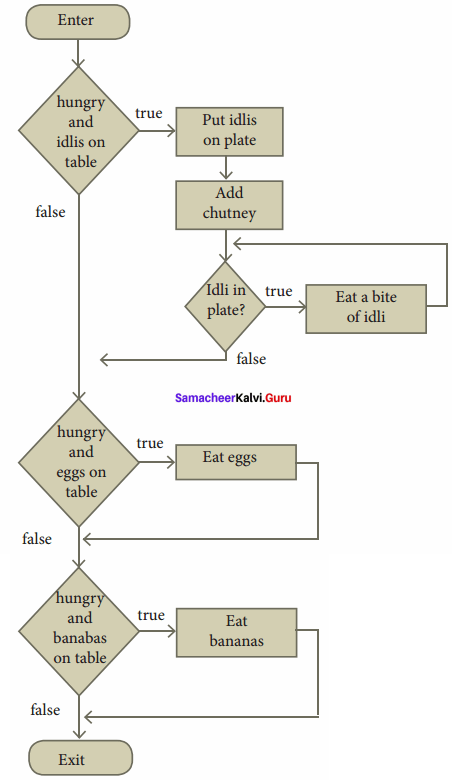Question 6.
Explain the Alternative statement.
Alternative statement:
A condition is a phrase that describes a test of the state. If C is a condition and both S1 and S2 are statements, then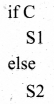is a statement, called an alternative statement, that describes the following action: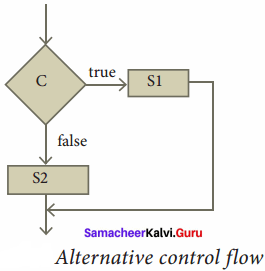1. Test whether C is true or false.
2. If C is true, then do S1; otherwise do S2.

In pseudo-code, the two alternatives S1 and S2 are indicated by indenting them from the keywords if and else, respectively. Alternative control flow is depicted in the flowchart. Condition C has two outgoing arrows, labeled true and false. The true arrow points to the S1 box. The false arrow points to the S2 box. Arrows out of S1 and S2 point to the same box, the box after the alternative statement.

Conditional statement:
Sometimes we need to execute a statement only if a condition is true and do nothing if the condition is false. This is equivalent to the alternative statement in which the else clause is empty. This variant of the alternative statement is called a conditional statement.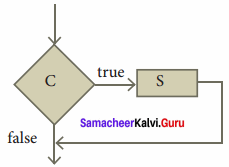If C is a condition and S is a statement, then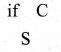1. Test whether C is true or false.
2. If C is true then do S; otherwise do nothing.

The conditional control flow is depicted in the flowchart.

Question 7.
Explain various symbols in the Flow chart.
A flowchart is a diagrammatic notation for representing algorithms. They show the control flow of algorithms using diagrams in a visual manner. In flowcharts, rectangular boxes represent simple statements, diamond-shaped boxes represent conditions, and arrows describe how the control flows during the execution of the algorithm. A flowchart is a collection of boxes containing statements and conditions which are connected by arrows showing the order in which the boxes are to be executed.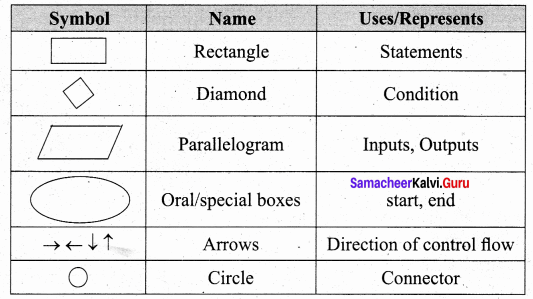1. A statement is contained in a rectangular box with a single outgoing arrow, which points to the box to be executed next.
2. A condition is contained in a diamond-shaped box with two outgoing arrows, labeled true and false. The true arrow points to the box to be executed next if the condition is true, and the false arrow points to the box to be executed next if the condition is false.
3. Parallelogram boxes represent inputs given and outputs produced.
4. Special boxes marked Start and the End is used to indicate the start and the end of execution:

The flowchart of an algorithm to compute the quotient and remainder after dividing an integer A by another integer B is shown below, illustrating the different boxes such as input, output, condition, and assignment, and the control flow between the boxes.

1. Flowcharts are less compact than the representation of algorithms in a programming language or pseudo code.
2. They obscure the basic hierarchical structure of the algorithms.
3. Alternative statements and loops are disciplined control flow structures.
4. Flowcharts do not restrict us to disciplined control flow structures.

## How to Prepare using Samacheer Kalvi 11th Computer Science Chapter 7 Composition and Decomposition Notes PDF?

Students must prepare for the upcoming exams from Samacheer Kalvi 11th Computer Science Chapter 7 Composition and Decomposition Notes PDF by following certain essential steps which are provided below.

• Use Samacheer Kalvi 11th Computer Science Chapter 7 Composition and Decomposition notes by paying attention to facts and ideas.
• Pay attention to the important topics
• Refer TN Board books as well as the books recommended.
• Correctly follow the notes to reduce the number of questions being answered in the exam incorrectly
• Highlight and explain the concepts in details.

## Frequently Asked Questions on Samacheer Kalvi 11th Computer Science Chapter 7 Composition and Decomposition Notes

#### How to use Samacheer Kalvi 11th Computer Science Chapter 7 Composition and Decomposition Notes for preparation??

Read TN Board thoroughly, make separate notes for points you forget, formulae, reactions, diagrams. Highlight important points in the book itself and make use of the space provided in the margin to jot down other important points on the same topic from different sources.

#### How to make notes for Samacheer Kalvi 11th Computer Science Chapter 7 Composition and Decomposition exam?

Read from hand-made notes prepared after understanding concepts, refrain from replicating from the textbook. Use highlighters for important points. Revise from these notes regularly and formulate your own tricks, shortcuts and mnemonics, mappings etc.
Share: